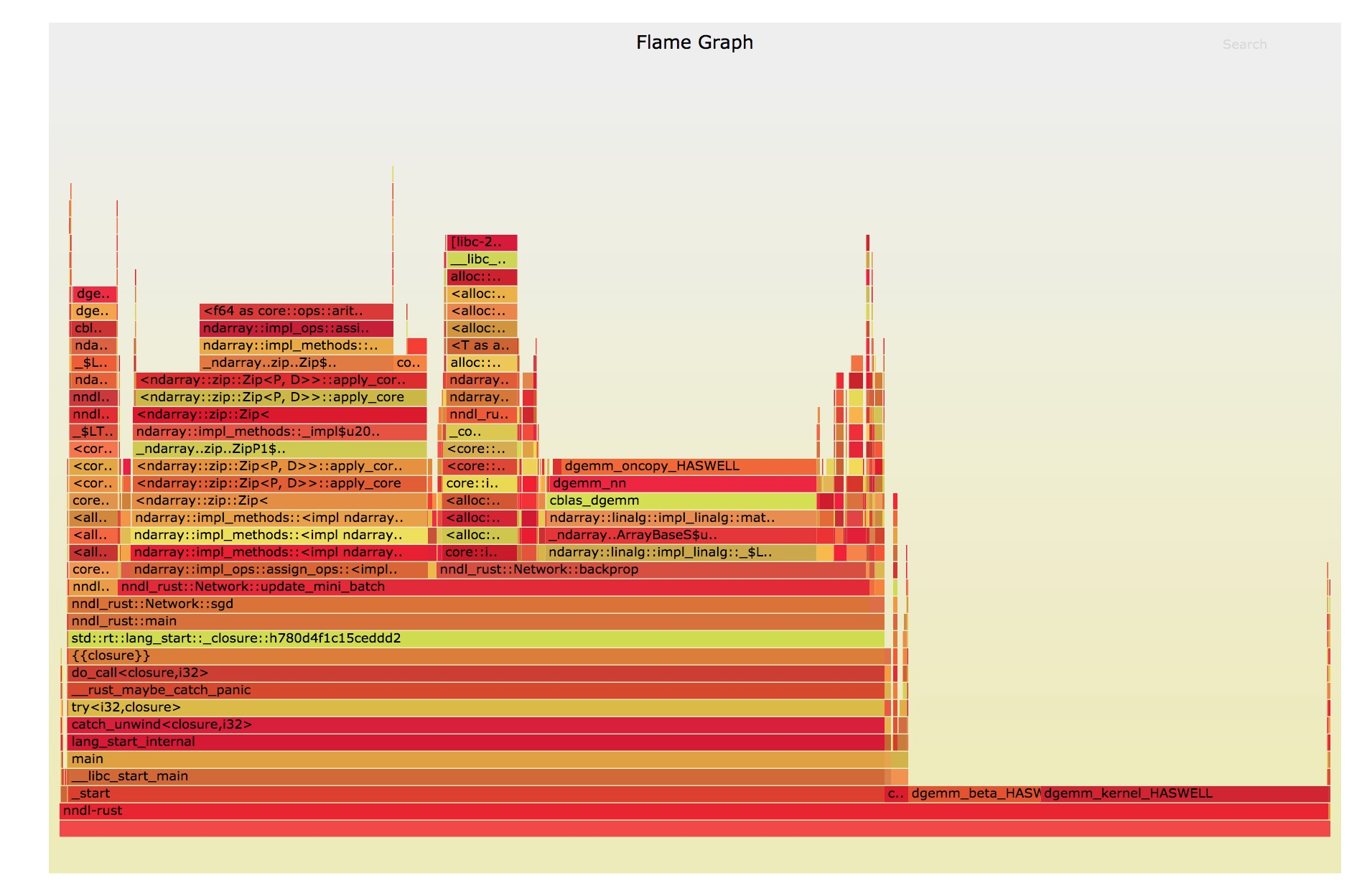# 在数据科学领域，Rust 会是 Python 的最佳替代方案吗？## Python 实现

class Network(object):     def __init__(self, sizes):        """The list sizes contains the number of neurons in the        respective layers of the network.  For example, if the list        was [2, 3, 1] then it would be a three-layer network, with the        first layer containing 2 neurons, the second layer 3 neurons,        and the third layer 1 neuron.  The biases and weights for the        network are initialized randomly, using a Gaussian        distribution with mean 0, and variance 1.  Note that the first        layer is assumed to be an input layer, and by convention we        won't set any biases for those neurons, since biases are only        ever used in computing the outputs from later layers."""        self.num_layers = len(sizes)        self.sizes = sizes        self.biases = [np.random.randn(y, 1) for y in sizes[1:]]        self.weights = [np.random.randn(y, x)                        for x, y in zip(sizes[:-1], sizes[1:])]

Network 类公开了两个可以被用户直接调用的方法。第一个方法是 evaluate 方法，这个方法可以通过网络尝试识别一系列测试图片中的数字，然后基于先验已知的正确结果，对识别的结果进行打分。第二个方法是 SGD 方法，这个方法可以通过遍历一组图片来执行随机梯度下降的过程。这个过程包括：将整组图像分解成小的类别，基于各个小类别图像来更新网络状态，更新用户指定的学习率，eta，以及在用户随机指定数量的一系列小类别图像上重新运行以上的训练步骤。每组小分类图像和网络的更新的核心算法如下代码所示：

def update_mini_batch(self, mini_batch, eta):    """Update the network's weights and biases by applying    gradient descent using backpropagation to a single mini batch.    The mini_batch is a list of tuples (x, y), and eta    is the learning rate."""    nabla_b = [np.zeros(b.shape) for b in self.biases]    nabla_w = [np.zeros(w.shape) for w in self.weights]    for x, y in mini_batch:        delta_nabla_b, delta_nabla_w = self.backprop(x, y)        nabla_b = [nb+dnb for nb, dnb in zip(nabla_b, delta_nabla_b)]        nabla_w = [nw+dnw for nw, dnw in zip(nabla_w, delta_nabla_w)]    self.weights = [w-(eta/len(mini_batch))*nw                    for w, nw in zip(self.weights, nabla_w)]    self.biases = [b-(eta/len(mini_batch))*nb                   for b, nb in zip(self.biases, nabla_b)]

## Rust 实现

use ndarray::Array2; #[derive(Debug)]struct Network {    num_layers: usize,    sizes: Vec<usize>,    biases: Vec<Array2<f64>>,    weights: Vec<Array2<f64>>,}

use rand::distributions::StandardNormal;use ndarray::{Array, Array2};use ndarray_rand::RandomExt; impl Network {       fn new(sizes: &[usize]) -> Network {        let num_layers = sizes.len();        let mut biases: Vec<Array2<f64>> = Vec::new();        let mut weights: Vec<Array2<f64>> = Vec::new();        for i in 1..num_layers {            biases.push(Array::random((sizes[i], 1), StandardNormal));            weights.push(Array::random((sizes[i], sizes[i - 1]), StandardNormal));        }        Network {            num_layers: num_layers,            sizes: sizes.to_owned(),            biases: biases,            weights: weights,        }    } }

## 类型和所有权

impl Network {    fn update_mini_batch(        &mut self,        training_data: &[MnistImage],        mini_batch_indices: &[usize],        eta: f64,    ) {        let mut nabla_b: Vec<Array2<f64>> = zero_vec_like(&self.biases);        let mut nabla_w: Vec<Array2<f64>> = zero_vec_like(&self.weights);        for i in mini_batch_indices {            let (delta_nabla_b, delta_nabla_w) = self.backprop(&training_data[*i]);            for (nb, dnb) in nabla_b.iter_mut().zip(delta_nabla_b.iter()) {                *nb += dnb;            }            for (nw, dnw) in nabla_w.iter_mut().zip(delta_nabla_w.iter()) {                *nw += dnw;            }        }        let nbatch = mini_batch_indices.len() as f64;        for (w, nw) in self.weights.iter_mut().zip(nabla_w.iter()) {            *w -= &nw.mapv(|x| x * eta / nbatch);        }        for (b, nb) in self.biases.iter_mut().zip(nabla_b.iter()) {            *b -= &nb.mapv(|x| x * eta / nbatch);        }    }}

fn to_tuple(inp: &[usize]) -> (usize, usize) {    match inp {        [a, b] => (*a, *b),        _ => panic!(),    }} fn zero_vec_like(inp: &[Array2<f64>]) -> Vec<Array2<f64>> {    inp.iter()        .map(|x| Array2::zeros(to_tuple(x.shape())))        .collect()}

let (delta_nabla_b, delta_nabla_w) = self.backprop(&training_data[*i]);

for (nb, dnb) in nabla_b.iter_mut().zip(delta_nabla_b.iter()) {    *nb += dnb;}for (nw, dnw) in nabla_w.iter_mut().zip(delta_nabla_w.iter()) {    *nw += dnw;}

let nbatch = mini_batch_indices.len() as f64;for (w, nw) in self.weights.iter_mut().zip(nabla_w.iter()) {    *w -= &nw.mapv(|x| x * eta / nbatch);}for (b, nb) in self.biases.iter_mut().zip(nabla_b.iter()) {    *b -= &nb.mapv(|x| x * eta / nbatch);}

## 用 Rust 重写这些代码，然后一切都会好起来